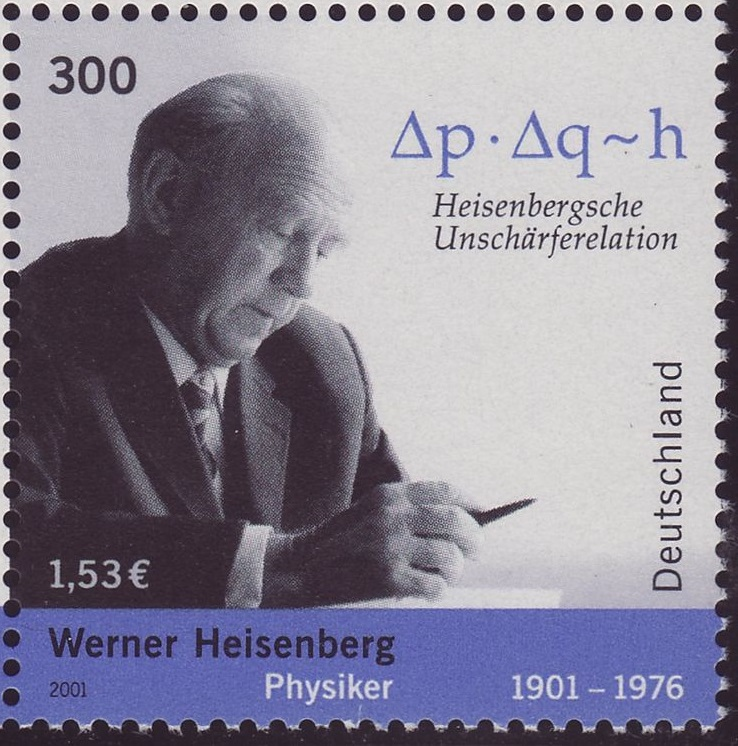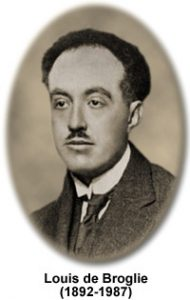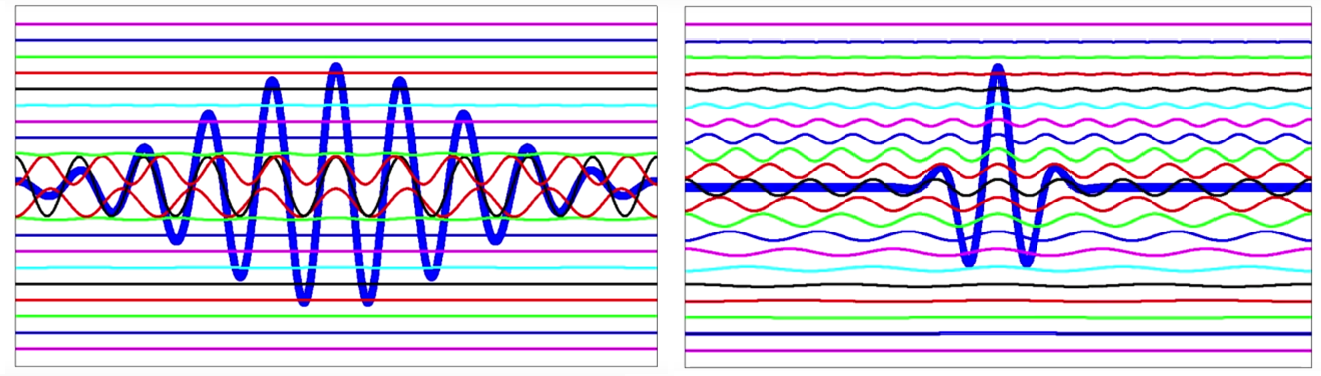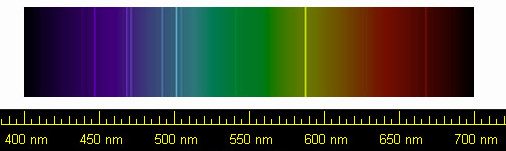4 Rates

# Heisenberg’s uncertainty principleFigure 1: Werner Heisenberg and the uncertainty principle. Source

Heisenberg’s uncertainty principle is one of the cornerstones of quantum physics. It is often misunderstood as the inability to exactly measure certain properties of quantum objects. In fact, it is an inherent property of nature itself. This article focuses on the meaning and effects of Heisenberg’s uncertainty principle.

In 1927 Werner Heisenberg discovered the uncertainty principle (Figure 1). This principle states that for certain value pairs of quantum objects both values cannot be exactly defined at the same time. The most well-known pair is position and momentum (momentum being the mass times the speed of a particle). Quantum particles therefore cannot have an exactly specified speed and exactly specified position at the same time. As a consequence there also cannot be an exactly specified particle path. Another such value pair – there are many others – is energy and time. Therefore, it is impossible to state that a quantum particle has exactly energy Y at point in time X.Figure 2: Louis de Broglie Source

One analogy to help understand the uncertainty principle comes from music: In order to determine the exact pitch of a guitar string the string must vibrate long enough to allow the measurement of the duration of the oscillation period. However, if this was measured over a certain period of time, it would be impossible to define the exact time of the measurement. This means the exact point in time of the measurement and the exact pitch are mutually exclusive. This is the uncertainty principle at work in the field of music. However, as quantum mechanics describes particles as waves, the relevance of this analogy can be seen shortly.

Although the waves which describe quantum particles are abstract, they still have all the typical properties of waves: they can add up, cancel one another out and more. Louis de Broglie (Figure 2) postulated in 1924 that each particle has a wavelength which is inversely proportional to its momentum. A fast, heavy particle therefore has a very short wavelength; a slow, light particle has a much longer wavelength. For example, the wavelength of fast electrons is considerably smaller than the diameter of an atom. Today, it is therefore possible to depict nanometer-sized samples at the atomic level using the best electron microscopes (see here).

## Position-momentum uncertainty

An exact position for a particle is described by a wave packet which only shows a high amplitude at this position and vanishes elsewhere. A wave packet comprises a higher number of waves of different wavelengths the narrower it is. Figure 3 shows on the left a wide wave packet which comprises a few waves of different wavelengths. To the right is a considerably narrower wave packet which contains many more waves of different wavelengths. It is easy to imagine that a very narrow wave packet must consist of an almost infinite number of different wavelengths.Figure 3: Wave packets consisting of waves with different wavelengths Source

A narrow wave packet therefore consists of many waves with different wavelengths. Consequently, the momentum – which is inversely proportional to the wavelength – has no sharply defined value but a wide distribution. For measurements this means that we measure very different momentum values despite identical preparation of the particles. We can summarize this as follows: If the position of a quantum object is exactly defined its momentum and speed must be undetermined and vice versa.

This has many consequences: Atoms are approximately 0.1 nanometers in size, which means that their electrons are limited to this space. It follows that the uncertainty of the speed of the electrons is in the order of magnitude of 1000 kilometers per second. Electrons can therefore have no defined orbits. Instead they form standing waves around the atomic nucleus. These standing waves are called orbitals. The absolute square value of the wave amplitude at each position gives the probability of finding the electron in this position.

If the atom was to be squeezed down to one tenth of its original size this would mean that the momentum of the electron would increase ten-fold and its energy would increase approximately one-hundred-fold. This amount of energy would need to be applied to the atom in order to squeeze it down. This is not possible under normal conditions on Earth, thus explaining the stability of the atoms. However, on neutron stars – these are burnt out stars with a remaining mass in the order of magnitude of our sun, but with a diameter of only approximately 30 kilometers – the force of gravity is so strong that the electrons are squeezed into the nucleus. A piece of neutron star the size of a sugar cube therefore weighs several hundred million tons.

With light-based analytical instruments the designers of the optics have to struggle with the uncertainty principle. The more they limit the diameter of a light beam, the more the position of the photons becomes exactly defined perpendicular to the beam direction. Therefore their momentum perpendicular to the beam direction becomes increasingly less defined. This has consequences: If a light beam is shone through an aperture with a diameter of a tenth of a millimeter, the diameter of the beam one meter behind the opening is already 10 millimeters, one hundred times larger than the opening itself. This effect, however, is not always a problem. It can be used to determine the dimensions of the structures which restrict the beam.

## Time-energy uncertainty

Whereas in the previous section for Figure 3 discussed the spatial dimension in the horizontal direction, now the dimension of time is discussed. Figuratively speaking we observe the wave packet through a narrow gap and see how it moves past us and record the height of the amplitude over time. The following is true: the shorter the wave packet, the more waves of different frequencies it contains. According to Max Planck the energy is proportional to the frequency. Therefore, a wave packet which is short in time has a wide energy distribution. This explains the time-energy uncertainty.

The time-energy uncertainty allows particles to borrow energy from nature as long as they restore this energy quickly enough. This allows them to overcome barriers which require more kinetic energy than they actually have. This so-called tunnel effect is what makes nuclear fusion in the sun possible, creating heat and light.Figure 4: Spectral lines of helium gas in visible light Source

The time-energy uncertainty is also found in spectroscopy. It is well-known that electrons in atoms and molecules have a ground state with minimum energy and excited states with higher levels of energy. These energy levels correspond with the previously mentioned orbitals. When moving from one state to another the energy difference is absorbed or radiated as photons with characteristic wavelengths. In spectroscopy this is used to identify atoms or molecules (Figure 4) or to determine their concentrations. The spectral lines of these transitions are broadened by disturbances such as the thermal movement of atoms and molecules. However, even if we could remove all disturbances the spectral lines would not be completely sharp. As a result of the time-energy uncertainty the spectral lines show the natural line width. If the energy difference was exactly defined, the corresponding transition time would be infinitely large and therefore there would be no transitions between the states.

## Conclusion

Although Heisenberg’s uncertainty principle seems far removed from reality, the consequences affect many – if not all – parts of life. That it is possible to master its effects is demonstrated by products from Anton Paar. With Alcolyzer an alcohol meter using infrared spectroscopy, the spectral properties of beverages are resolved on the nanometer scale in order to determine the alcohol content accurately. The new SAXSpoint™ uses X-rays to investigate the dimensions of the outer and inner structures of materials on the nanometer scale. In both cases the dispersion of the photons resulting from the uncertainty principle helps to exactly determine the properties of samples. The uncertainty principle therefore allows us to gain a sharp picture of nature.